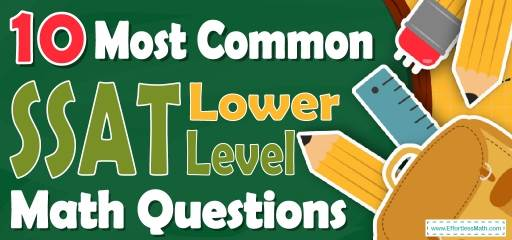# 10 Most Common SSAT LOWER LEVEL Math QuestionsPreparing for the SSAT LOWER LEVEL Math test? Want a preview of the most common mathematics questions on the SSAT LOWER LEVEL Math test? If so, then you are in the right place.

The mathematics section of SSAT LOWER LEVEL can be a challenging area for many test-takers, but with enough patience, it can be easy and even enjoyable!

Preparing for the SSAT LOWER LEVEL Math test can be a nerve-wracking experience. Learning more about what you’re going to see when you take the SSAT LOWER LEVEL can help to reduce those pre-test jitters. Here’s your chance to review the 10 most common SSAT LOWER LEVEL Math questions to help you know what to expect and what to practice most. Try these 10 most common SSAT LOWER LEVEL Math questions to hone your mathematical skills and to see if your math skills are up to date on what’s being asked on the exam or if you still need more practice.

Make sure to follow some of the related links at the bottom of this post to get a better idea of what kind of mathematics questions you need to practice.

## 10 Sample SSAT LOWER LEVEL Math Practice Questions

1- If 5 is added to a number, the sum is 20. If the same number is added to 25, the answer is

A. 35

B. 30

C. 45

D. 40

E. 55

2- $$\frac{2+5+6×1+1}{3+5} =?$$

A. $$\frac{15}{8}$$

B. $$\frac{4}{8}$$

C. $$\frac{7}{4}$$

D. $$\frac{6}{8}$$

E. $$\frac{10}{8}$$

3- What is the Area of the square shown in the following square?

A. 2

B. 4

C. 6

D. 8

E. 10

4- If 20 can be divided by both 4 and? without leaving a remainder, then 20 can also be divided by which of the following?

A. $$x+4$$

B. $$x? -4$$

C. $$x-2$$

D. $$x×4$$

E. $$x+1$$

5- Use the equations below to answer the question:
$$x−5=1$$
$$3+y=8$$
What is the value of $$(x+y)$$?

A. 9

B. 10

C. 11

D. 12

E. 14

6- Which of the following expressions has the same value as $$\frac{5}{4}×\frac{6}{2}$$?

A. $$\frac{6×3}{4}$$

B. $$\frac{6×2}{4}$$

C. $$\frac{5×6}{4}$$

D. $$\frac{5×3}{4}$$

E. $$\frac{8×3}{4}$$

7- When 5 is added to three times number N, the result is 41. Then N is ….

A. 11

B. 12

C. 14

D. 16

E. 18

8- At noon, the temperature was 15 degrees. By midnight, it had dropped another 20 degrees. What was the temperature at midnight?

A. 10 degrees above zero

B. 10 degrees below zero

C. 5 degrees above zero

D. 5 degrees below zero

E. 15 degrees below zero

9- If a triangle has a base of 5 cm and a height of 8 cm, what is the area of the triangle?

A. 15cm$$^2$$

B. 20cm$$^2$$

C. 40cm$$^2$$

D. 45cm$$^2$$

E. 50cm$$^2$$

10- Which formula would you use to find the area of a square?

A.

B.

C.

D.

E.

## Best SSAT LOWER LEVEL Math Prep Resource for 2022

1- D
$$5+x=20→x=15→15+25=40$$

2- C
$$\frac{2+5+6×1+1}{3+5} =\frac{14}{8}=\frac{7}{4}$$

3- B
Area of a square $$=$$ (one side) $$×$$ (one side)
$$2×2=4$$

4- D
$$20=x×4→x=20÷4=5$$
$$x$$ equals to 5. Let’s review the options provided:
A) $$x+4→5+4=9$$
20 is not divisible by 9.
B) $$2x-4→2×5-4=6$$
20 is not divisible by 6.
C) $$x-2→5-2=3$$ 20
is not divisible by 3.
D) $$x×4→5×4=20$$
20 is divisible by 20.
E) $$x+1→5+1=6$$
20 is not divisible by 6.

5- C
$$x-5=1→x=6$$
$$3+y=8→y=5$$
$$x+y=6+5=11$$

6- D
$$\frac{5}{4}×\frac{6}{2}=\frac{30}{8}=\frac{15}{4}$$
Choice D is equal to $$\frac{15}{4}$$.
$$\frac{(5×3)}{4}=\frac{15}{4}$$

7- B
$$5+3N=41→3N=41-5=36→N=12$$

8- D
$$15-20=-5$$
The temperature at midnight was 5 degrees below zero.

9- B
Area of a triangle = $$\frac{1}{2}×(base)×(height)= \frac{1}{2}×5×8=20$$

10- D
$$area \ of \ a \ square=side×side$$

Looking for the best resource to help you succeed on the SSAT LOWER LEVEL Math test?

## The Best Books to Ace the SSAT LOWER LEVEL Math Test

### What people say about "10 Most Common SSAT LOWER LEVEL Math Questions - Effortless Math: We Help Students Learn to LOVE Mathematics"?

No one replied yet.

X
30% OFF

Limited time only!

Save Over 30%

SAVE $5 It was$16.99 now it is \$11.99